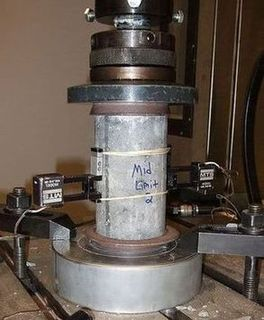# Concrete compressive strength:7,28 daysConcrete compressive strength: For determining the characteristic compressive strength of concrete cube tests is done. Specimens are used for Concrete testing.

## What is the Concrete compressive strength after 7 days and 28 days?

The characteristic compressive strength of concrete is determined by performing cube tests and they are performed utilising concrete specimens of a cubical mould of size 15 cm x 15 cm x 15 cm and the cylindrical form of dimension 15 cm in diameter x 30 cm in height.
The compressive strength test of the concrete cylindrical specimen is 0.8 times the strength of cube.
Concrete compression test of specimens is done after 28 days of curing.
With the increase in age, the strength of concrete will increase.
concrete compressive strength at any age = Strength at 28 days x age factor.
Age factors  at different age of concrete

 S.No Minimum age Age factor 1 7 days 0.7 2 28 days 1.0 3 3 months 1.10 4 6 months 1.15 5 12 months 1.2

For example,

concrete Compressive strength of M 25 grade of concrete at 28 days in 25 N/mm2.
So concrete compressive strength of M 25 grade concrete at 7 days = 0.7 x 25= 17.5 N/mm2.
Note: By using High Alumina cement concrete, the strength of concrete will not increase with time.

## Properties of Reinforcement Cement Concrete

Apart from the concrete compressive strength, given below other properties of reinforcement concrete which are related to the compressive strength of concrete.
1. Tensile strength of concrete
2. Elastic deformation
3. Shrinkage
4. Creep of concrete
5. Workability
6. Durability of concrete

### 1. Tensile strength of concrete

Tensile strength of concrete is determined by using the characteristic compressive strength of concrete.
Formula :
ft = 0.7 x square root of fck.

### 2. Elastic Deformation

The modulus of elasticity is principally controlled by the elastic properties of the aggregate and to a more secondary by mix proportions, the conditions of curing, the type of cement, age of the concrete.

#### 5 best methods of Concrete mix design

Modulus of elasticity is reliant on the concrete compressive strength.
Concrete modulus of elasticity can be determined utilising the formula
Ec = 5000 x square roof of fck  (new IS 2000),Ec is the Static modulus of elasticity

### 3. Shrinkage of concrete

The complete shrinkage of concrete depends on the environmental conditions, size of the member, constituents of concrete.
Total shrinkage of concrete is influenced by the total amount of water or moisture present in concrete.
Shrinkage strain in concrete = 0.0003.

### 4. Creep of concrete

Under suspended loading, if there is a continuous deformation of concrete with time then it is termed as the creep of concrete.
The coefficient of Creep   = Ultimate creep strain / elastic strain at the age of loading
Given below creep coefficient at different ages.
 S.No Age of loading Creep coefficient 1 7 days 2.2 2 28 days 1.6 3 1 year 1.1

### 5. Workability

Workability of concrete is the ease with which concrete can be placed and compacted.
Based on slump cone test values, degree of workability given below
 S.No Slump(mm) Vee-Bee time (sec) Compaction factor Degree of workability 1 0 – 25 25 – 10 0.75 – 0.8 Very low 2 25 – 50 10 – 5 0.8 – 0.85 Low 3 50 – 100 5 – 2 0.85 – 0.93 Medium 4 100 – 175 – > 0.92 High

### 6. Durability of concrete

Durability is the ability to last for long.
Durable concrete is the concrete when exposed to environmental conditions If it performs satisfactorily in the working.
To protect the embedded metal from corrosion, the factor of safety to be considered.

#### i.For ductile material like mild steel

the factor of safety value is given by  = Yield stress/Working stress
The factor of safety for steel =1.67
The factor of safety for RCC = 1.78

#### ii.For brittle material like concrete

F.O.S = Ultimate stress / working stress.
F.O.S in flexure = 3

### The factor of safety for RCC is more than Steel.

Graeter the factor of safety for RCC needs to be considered. Given the reasons below.
1. Concrete is a brittle material, the failure of a brittle material is sudden without any warning.
The hence higher factor of safety is considered for RCC.
2. Steel is manufacturing in a factory and its quality control is certified.
The casting of Concrete is in position and quality control is less than that of factory-made.
The hence higher factor of safety is considered for RCC.